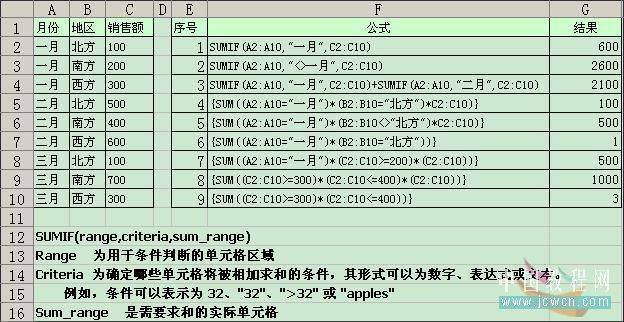# EXCEL 多条件求和

.中国教程网zhangditony翻译，转载请保留此信息 .

This tip provides a number of examples that should solve most of your counting and summing problems.
The example formulas presented in this tip use the simple database table shown below. You will need to adjust the formulas to        account for your own data.
1.Sum of Sales, where Month="Jan"--求一月销售额之和
This is a straightforward use of the SUMIF function (it uses a single criterion):
=SUMIF(A2:A10,"Jan",C2:C10)
2.Sum of Sales, where Month<>"Jan"--求一月之外月份的销售额之和
Another simple use of SUMIF (single criterion):
=SUMIF(A2:A10,"<>Jan",C2:C10)
3.Sum of Sales where Month="Jan" or "Feb"--求一月或二月销售额之和（公式中的加号表示“或”的意思）
For multiple OR criteria in the same field, use multiple SUMIF functions:
=SUMIF(A2:A10,"Jan",C2:C10)+SUMIF(A2:A10,"Feb",C2:C10)
4.Sum of Sales where Month="Jan" AND Region="North"--求一月并且地区为北方的销售额之和
For multiple criteria in different fields, the SUMIF function doesn't work. However, you can use an array formula. When you enter      this formula, use Ctrl+Shift+Enter:
=SUM((A2:A10="Jan")*(B2:B10="North")*C2:C10)(数组公式,输入公式后按ctrl+shift+enter)
5.Sum of Sales where Month="Jan" AND Region<>"North"--求一月并且北方之外地区的销售额之和
Requires an array formula similar to the previous formula. When you enter this formula, use Ctrl+Shift+Enter:
=SUM((A2:A10="Jan")*(B2:B10<>"North")*C2:C10)(数组公式)
6.Count of Sales where Month="Jan" AND Region="North"--计算一月并且地区为北方的数量For multiple criteria in different fields, the COUNTIF function doesn't work.  you can use an array formula.
=SUM((A2:A10="Jan")*(B2:B10="North"))(数组公式)
7.Sum of Sales where Month="Jan" AND Sales>= 200--求一月份销售额超过200的销售额之和
Requires an array formula similar to the previous example. When you enter this formula, use Ctrl+Shift+Enter:
=SUM((A2:A10="Jan")*(C2:C10>=200)*(C2:C10))(数组公式)
8.Sum of Sales between 300 and 400--求销售额在300和400之间的销售额之和
This also requires an array formula. When you enter this formula, use Ctrl+Shift+Enter:
=SUM((C2:C10>=300)*(C2:C10<=400)*(C2:C10))(数组公式)
9.Count of Sales between 300 and 400--计算销售额在300至400之间的数量
This also requires an array formula. When you enter this formula, use Ctrl+Shift+Enter:
=SUM((C2:C10>=300)*(C2:C10<=400))(数组公式)App下载